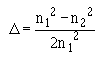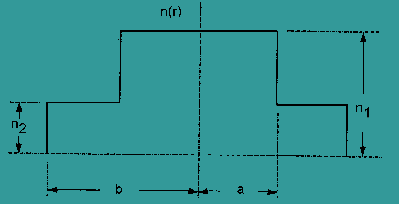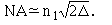Custom SearchMULTIMODE STEP-INDEX FIBERS A multimode step-index fiber has a core of radius (a) and a constant refractive index n1. A cladding of slightly lower refractive index n2 surrounds the core. Figure 3-2 shows the refractive index profile n(r) for this type of fiber. n(r) is equal to n1 at radial distances r < a (core). n(r) is equal to n2 at radial distances r ≥ a (cladding). Notice the step decrease in the value of refractive index at the core-cladding interface. This step decrease occurs at a radius equal to distance (a). The difference in the core and cladding refractive index is the parameter Δ:Δ is the relative refractive index difference. Figure 3-2. - The refractive index profile for multimode step-index fibers.The ability of the fiber to accept optical energy from a light source is related to Δ. Δ also relates to the numerical aperture byThe number of modes that multimode step-index fibers propagate depends on Δ and core radius (a) of the fiber. The number of propagating modes also depends on the wavelength (λ) of the transmitted light. In a typical multimode step-index fiber, there are hundreds of propagating modes. Most modes in multimode step-index fibers propagate far from cutoff. Modes that are cut off cease to be bound to the core of the fiber. Modes that are farther away from the cutoff wavelength concentrate most of their light energy into the fiber core. Modes that propagate close to cutoff have a greater percentage of their light energy propagate in the cladding. Since most modes propagate far from cutoff, the majority of light propagates in the fiber core. Therefore, in multimode step-index fibers, cladding properties, such as cladding diameter, have limited affect on mode (light) propagation. Multimode step-index fibers have relatively large core diameters and large numerical apertures. A large core size and a large numerical aperture make it easier to couple light from a light-emitting diode (LED) into the fiber. Multimode step-index fiber core size is typically 50 μm or 100 μm. Unfortunately, multimode step-index fibers have limited bandwidth capabilities. Dispersion, mainly modal dispersion, limits the bandwidth or information-carrying capacity of the fiber. System designers consider each factor when selecting an appropriate fiber for each particular application. Multimode step-index fiber selection depends on system application and design. Short-haul, limited bandwidth, low-cost applications typically use multimode step-index fibers. Q.5 Multimode step-index fibers have a core and cladding of constant refractive index n1 and n2, respectively. Which refractive index, the core or cladding, is lower? Q.6 In multimode step-index fibers, the majority of light propagates in the fiber core for what reason? Q.7 Multimode step-index fibers have relatively large core diameters and large numerical apertures. These provide what benefit?